# Test: Vector (Competition Level) - 1

## 30 Questions MCQ Test Mathematics (Maths) Class 12 | Test: Vector (Competition Level) - 1

Description
This mock test of Test: Vector (Competition Level) - 1 for JEE helps you for every JEE entrance exam. This contains 30 Multiple Choice Questions for JEE Test: Vector (Competition Level) - 1 (mcq) to study with solutions a complete question bank. The solved questions answers in this Test: Vector (Competition Level) - 1 quiz give you a good mix of easy questions and tough questions. JEE students definitely take this Test: Vector (Competition Level) - 1 exercise for a better result in the exam. You can find other Test: Vector (Competition Level) - 1 extra questions, long questions & short questions for JEE on EduRev as well by searching above.
QUESTION: 1

### If the vector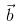is collinear with the vector (2√2, -14) and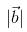= 10, then

Solution:

a = 2(2)½ i -  j + 4k
|b| = 10
[(2(2λ)2 + (1λ)2 + (4λ)2]½
=[ 8λ2 + λ2 + 16λ2]½ = 10
= 25λ2 = (10)2
25λ2 = 100
λ = +-2
b = +-(4(2)½ i - 2j + 8k)
Therefore, 2a +- b

QUESTION: 2

### The vertices of a triangle are A(1, 1, 2), B(4, 3, 1) and C(2, 3, 5). A vector representing the internal bisector of the angle A is

Solution:

Let AD is the bisector of ∠A.Then,
BD/DC = AB/ACeq(1)
Given the vertices of triangle,
AB = √32 + 22 + 12 = √14
AC = √12 + 22 + 32 = √14
As AB = BC, So, BD = DC(from eq(1))
It means D is middle point of BC. So, vertices of D will be (3,3,3).
So, vector AD will be 2iˆ+ 2jˆ+ kˆ.

QUESTION: 3

### Let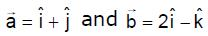. The point of intersection of lines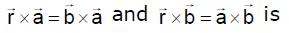Solution:
QUESTION: 4

If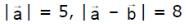and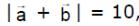then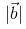is equal to

Solution:
QUESTION: 5

Angle between diagonals of a parallelogram whose side are represented by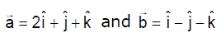Solution:

D1 = a+b
D2 = a-b
D1 = 3i + 0j + 0k
D2 = i + 2j + 2k
|D1| = 3
D1.D2 = |D1| . |D2| . cos θ
3 + 0 + 0 = (3) . (3) cos θ
3 = 9 cosθ
cos-1 = (⅓)

QUESTION: 6

Vector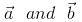make an angle θ = 2π/3. if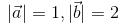, then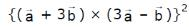is equal to

Solution: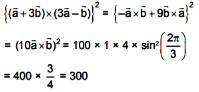QUESTION: 7

Unit vector perpendicular to the plane of the triangle ABC with position vectors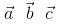of the vertices
A, B, C is

Solution:

Δ = 1/2(a * b) = 1/2(b*c) = 1/2(c*a)
2Δ = a*b = b*c = c*a
unit vector = 1/2Δ[a*b + b*c + c*a]

QUESTION: 8

The value of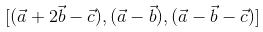is equal to the box product

Solution:

Matrix {(1,2,-1) (1,-1,0) (1,-1,-1)} [a b c]
= {1(1) -2(-1) -1(0)} [a b c]
= 3[a b c]

QUESTION: 9

If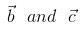are two non-collinear vectors such that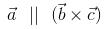, then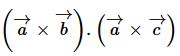is equal to

Solution:
QUESTION: 10

Vector of length 3 unit which is perpendicular to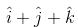and lies in the plane ofand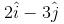Solution: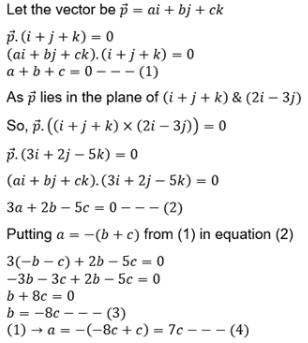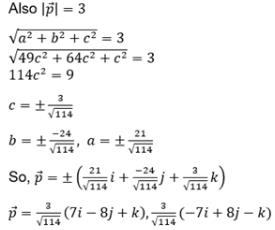QUESTION: 11

Vector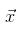satisfying the relation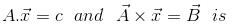Solution:

A * X = B
(A * X)*A = B * A
=> -A(x.A) + x(A.A) = B * A
=> -Ac + x|A|2 = B * A
x|A|2 = B * A + Ac
x = [B * A + Ac]/|A|2

QUESTION: 12

If a ,b,c are linearly independent vectors, then which one of the following set of vectors is linearly dependent ?

Solution:

xa + yb + zc = 0
We have to prove x = y = z = 0
a,b,c are non planner
x(a-b) +y(b-c) +c(c-a) = 0
Let x = 1, y = 1, z = 1
So, we get a - b + b - c + c - a = 0

QUESTION: 13

Let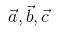be vectors of length 3,4,5 respectively. Let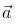be perpendicular to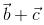,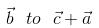and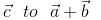. then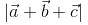Solution:

|A| = 3, |B| = 4, |C| = 5
Since A.(B + C) = B.(C+A) = C(A+B) = 0...........(1)
|A+B+C|2 = |A| + |B|2 + |C|2 + 2(A.B + B+C + C.A)
= 9+16+25+0
from eq(1) {A.B + B+C + C.A = 0}
therefore, |A+B+C|2 = 50
=> |A+B+C| = 5(2)1/2

QUESTION: 14

Given the vertices A (2, 3, 1), B(4, 1, –2), C(6, 3, 7) & D(–5, –4, 8) of a tetrahedron. The length of the altitude drawn from the vertex D is

Solution:
QUESTION: 15

for a non zero vector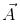If the equations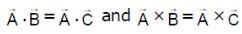hold simultaneously, then

Solution:
QUESTION: 16

The volume of the parallelopiped constructed on the diagonals of the faces of the given rectangular parallelopiped is m times the volume of the given parallelopiped. Then m is equal to

Solution:

Vi=[a→,b→,c→]
(a→ + b→)(b→ + c→)(a→ + c→)
Vf=[(a→ + b→)(b→ + c→)(a→ + c→)]
=(a→ + b→)⋅[(b→ + c→)⋅(a→ + c→)]
=(a→ +b→)[b→⋅a→ + c→⋅a→ + b→⋅c→ + c→⋅c→]
=[b→ c→ a→]+[a→ b→ c→]
Vf=2[a→ b→ c→]
Vf=2Vi.

QUESTION: 17

If u and v are unit vectors and θ is the acute angle between them, then 2u × 3v is a unit vector for

Solution:
QUESTION: 18

The value of a, for which the points A,B,C with position vectors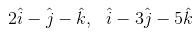and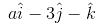respectively are the vertices of a right angled triangle with C = π/2 are

Solution: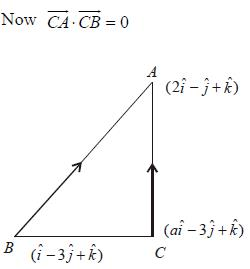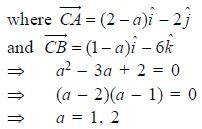QUESTION: 19

The distance between the line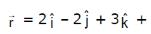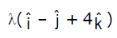and the plane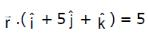is

Solution:
QUESTION: 20

A particle is acted upon by constant forces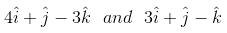which displace ot from a point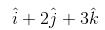to the point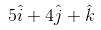. The workdone in standard units by the force is given by

Solution:
QUESTION: 21

If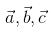are non-coplaner vectors and λ is a real number, then the vectors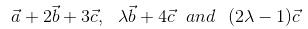are non-coplaner for

Solution:
QUESTION: 22

Let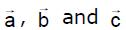be non zero vectors such that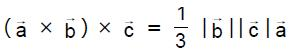, If θ is the acute angle between the vectors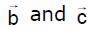, then sin θ equals is

Solution:

(a→*b→)*c→ = (1/3)|b→||c→||a→|
⇒ − c→*(a→*b→) = (1/3)|b→||c→||a→|
⇒(c→.a→)b→ −(c→.b→)a→ =(1/3)|b→||c→|a→|
Now, as all of them are non-collinear, (c→⋅a→) can be 0 that means,
(c→.b→) = (−1/3)|b→||c→|
⇒|b→|.|c→|cosθ = (−1/3)|b→||c→|
⇒ cosθ = −1/3
sinθ = √1−(1/3)^2
= √8/9
= (2√2)/√3

QUESTION: 23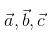are three vectors, such that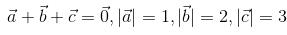then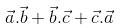is equal to

Solution:
QUESTION: 24

If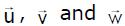are three non-coplaner vectors, then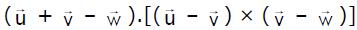equals

Solution:
QUESTION: 25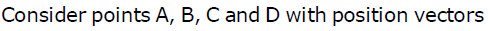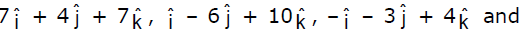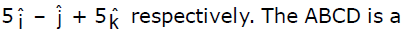Solution:
QUESTION: 26

The vectors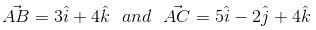are the sides of a triangle ABC. The length of the median through A is

Solution:

The length of median through A = vector(AB + BC)/2
= (3i + 4k + 5i - 2j + 4k)/2
= (8i - 2j + 8k)/2
= 4i - j + 4k
Length  = √(16 + 1 + 16) = √33

QUESTION: 27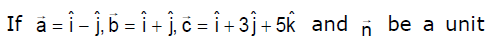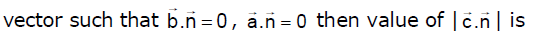Solution:
QUESTION: 28

If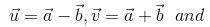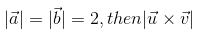is equal to

Solution:

∣u × v∣=∣(a − b) × (a + b)∣
=2∣a × b∣      (∵a × a = b × b = 0)
and ∣a × b∣2 + (a ⋅ b)2
=(ab sinθ)+ (abcosθ)2
=a2b2
⇒∣a×b∣ = a2b2−(a ⋅ b)2
So, ∣u × v∣ = 2∣a × b∣
=2[a ^ 2b− (a ⋅ b)2]1/2
=2[(2)2(2)2−(a⋅b)2]1/2
=2(16−(a⋅b)2)1/2
∴∣a∣=∣b∣=2

QUESTION: 29

Let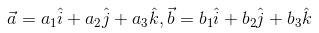and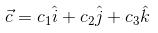be three non-zero vectors such that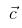is a unit vector perpendicular to both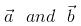. if the angle betweenis π/6, then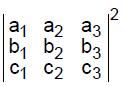is equal to

Solution:

According to the given conditions,
(c1)2+c(2)2+(c3)22 =1,a⋅c=0,b⋅c=0
and cosπ/6 = [(3)1/2]/2
​(a1b1+a2b2+a3b3]/[(a1)2+(a2)2 + (a3)^2)]^1/2 [(b1)^2 + (b2)2 + (b3)2]1/2
Thus a1c1+a2c2+a3c3=0, b1c1+b2c2+b3c3=0
and [(3)^1/2]/2[(a1)2+(a2)2 + (a3)2)1/2 ((b1)2 + (b2)2 +(b3)2]1/2
=a1b1+a2b2 +a3b3
[(a1)2+(a2)2 + (a3)2] -(a1b1 + a2b2 + a3b3)2
[(a1)2+(a2)2 + (a3)2] [(b1)2+(b2)2 + (b3)2]
= -3/4 [(a1)2+(a2)2 + (a3)2] [(b1)2+(b2)2 + (b3)2]
= 1/4 [(a1)2+(a2)2 + (a3)2] [(b1)2+(b2)2 + (b3)2]

QUESTION: 30

A point taken on each median of a triangle divides the median in the ratio 1 : 3, reckoning from the vertex.
Then the ratio of the area of the triangle with vertices at these points to that of the original triangle is

Solution:

Let A (0,0); B (4m , 0) and C(4p , 4q)
M1 (2m + 2p, 2q)
M2 (2p , 2q) and M3 (2m , 0)
Let E , F and G be the point on the median.
E = (2m + 2p) / 4, 2q / 4) = ((m + p) / 2, q / 2)
F = ((2p + 12m) / 4, (2q + 0) / 4) = ((p + 6m) / 2, q / 2)
G = ((2m + 12p) / 4, (0 + 12 q) / 4) = ((m + 6p ) / 2, 3q)
Area of traingle ABC = 1/2
Area of traingle ABC = 1/2 {(0,0,0) (4m,0,1) (4p,4q,1)}
=1/2(16) = 8 unit
Area of triangle EFG = 1/2 {((m + p) / 2, q / 2, 1)) ((p + 6m) / 2, q / 2, 1) ((m + 6p)/2, 3q, 1)}
= 25/8 unit
ar (EFG) /ar (ABC) = {25 / 8} / 8
= 25/64